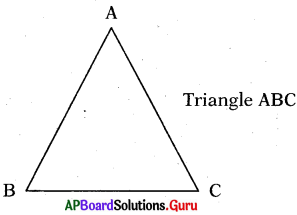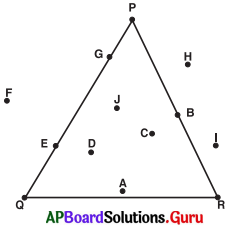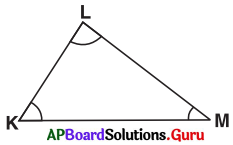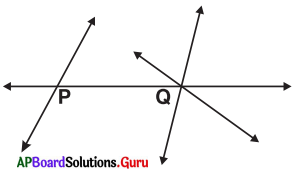SCERT AP 7th Class Maths Solutions Pdf Chapter 5 Triangles Review Exercise Questions and Answers.

## AP State Syllabus 7th Class Maths Solutions 5th Lesson Triangles Review Exercise

Question 1.
Mark any three non collinear points A, B and C in your note book, join them to make a triangle and name it.Question 2.
Observe the given triangle and answer the following :(i) Write the interior points of the triangle.
A, C, D and J.

(ii) Write the points marked on the triangle.
B, E, G, P, Q and R.

(iii) Write the exterior points of the triangle.
F, H and I.

Question 3.
Observe the given triangle and answer the following:(i) The opposite side to vertex L is __________
$$\overline{\mathrm{KM}}$$

(ii) The opposite side to ZK is __________
$$\overline{\mathrm{LM}}$$

(iii) The opposite angle to $$\overline{\mathrm{KL}}$$ is __________
∠M.

(iv) The opposite vertex to $$\overline{\mathrm{LM}}$$ is __________
K.Question 4.
Classify the following angles into acute, obtuse and right angles :
20°, 50°, 102°, 47°, 125°, 65°, 36°, 90°, 95° and 110°.